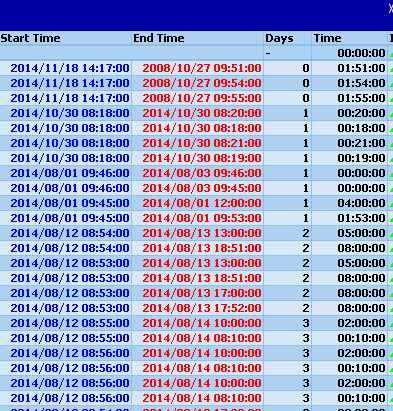# Qlik Education Discussions

Discussion Board for collaboration related to Qlik Education.Not applicable

## HElP: i need to calculate time spent on the system with the following start time and end time.7 Replies
Valued Contributor

## Re: HElP: i need to calculate time spent on the system with the following start time and end time.

Hello Kenneth,

You need to use the INTERVAL-function (see example below

*,
INTERVAL (End_time -Start_time , 'D hh:mm:ss') as interval;

@1 as Start_time,
@2 as End_time
FROM C:\Users\rfn7501\Documents\test.xls (biff, no labels, table is [Sheet1\$]);

Then, if you need days and hours separately, you go on with the interval field, using left and right functions.

Regards,Not applicable

## Re: HElP: i need to calculate time  spent on the system with the following start time and end time.

Dear Johan,

please advice on how can i use the left and right function because i need  days and time spent separately.

Thank you,

Kind Regards,

Kenneth

Valued Contributor

## Re: HElP: i need to calculate time spent on the system with the following start time and end time.

Hi Kenneth,

There's another way that is more sure, and that is Subfield. I've entered it into the example, so you can copy.

*,
Subfield (interval,' ',1) as Hour_difference,
Subfield (interval,' ',2) as Minutes_difference;

*,
INTERVAL(End_time-Start_time, 'D hh:mm:ss') as interval;

@1 as Start_time,
@2 as End_time
FROM

C:\Users\rfn7501\Documents\test.xls(biff, no labels, table is [Sheet1\$]);

What subfield does, is looking for a separator. In our case, this is a blank (' '), and then you define which part you need.

Regards,

Johan

Highlighted
Valued Contributor

## Re: HElP: i need to calculate time spent on the system with the following start time and end time.

Hello Kenneth,

Kind regards,

JohanNot applicable

## Re: HElP: i need to calculate time spent on the system with the following start time and end time.

Dear Johan,

im struggling on the subfield function ,I don't understand .what should I put within the ' ' (quotes).

or is there a way I can do this on the expression.

Thank you,

Kind regards,

Kenneth

Valued Contributor

## Re: HElP: i need to calculate time spent on the system with the following start time and end time.

Hello Kenneth,

If you take a look at the interval-function, then you'll see it is scripted 'D hh:mm:ss', where between D and hh, there is a space. This space you'll find back in your interval variable as well. (e.g. '2 00:12:12')

In order to separate the days (D) and the hours/minutes/seconds (hh:mm:ss), we will now use this space.

In the subfield-statement, you look for a unique separator, in this case the space, and you use that to 'cut' your variable.

Subfield (interval, ' ', 1) as Y actually reads like : take the variable interval, look for the separator 'space' (' '), and then return the first bit of information before the separator. So, between the brackets, you put a space (1 hit on your spacebar)

To make it more visible, you could script the interval function as 'D|hh:mm:ss'. The separator is then no longer a space, but a |.  Your variable becomes e.g. 2|00:12:12. The subfield command then becomes Subfield(interval, '|',1).

Regards,

JohanNot applicable

## Re: HElP: i need to calculate time  spent on the system with the following start time and end time.

Dear Johan,

Thank you,  i thought maybe i have to put something your help is much appreciated.

Kind regards,

Kenneth

Community Browser